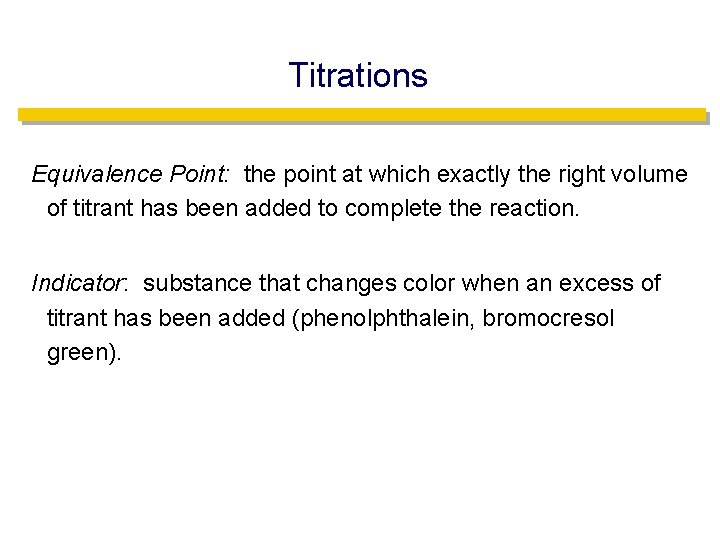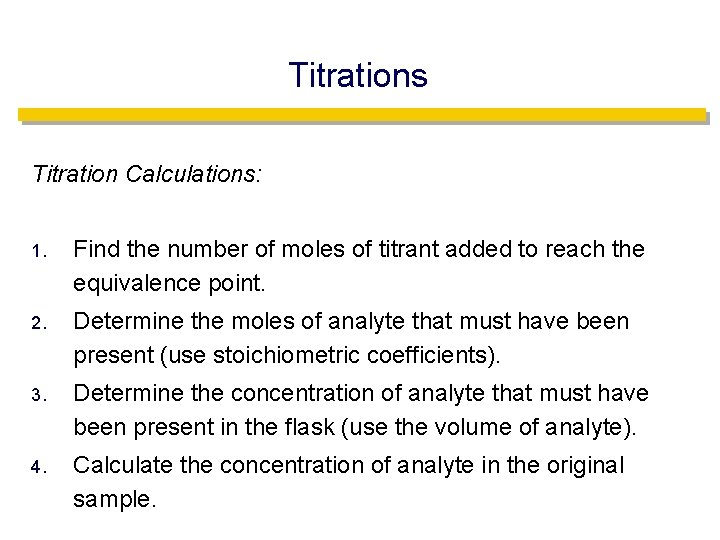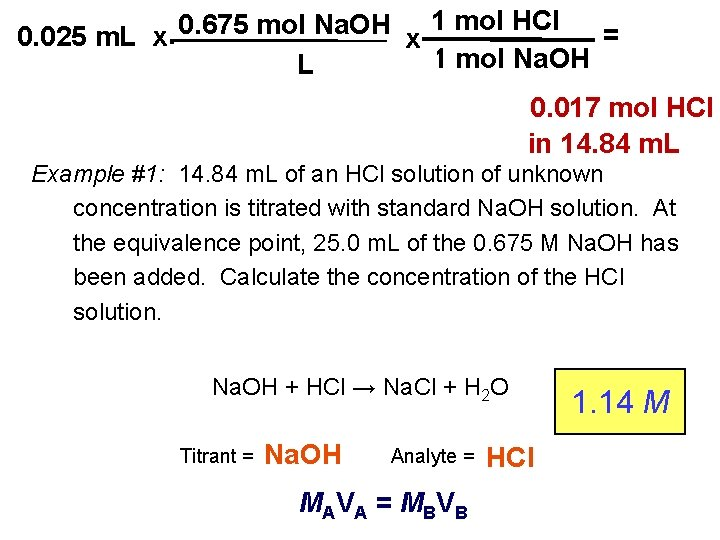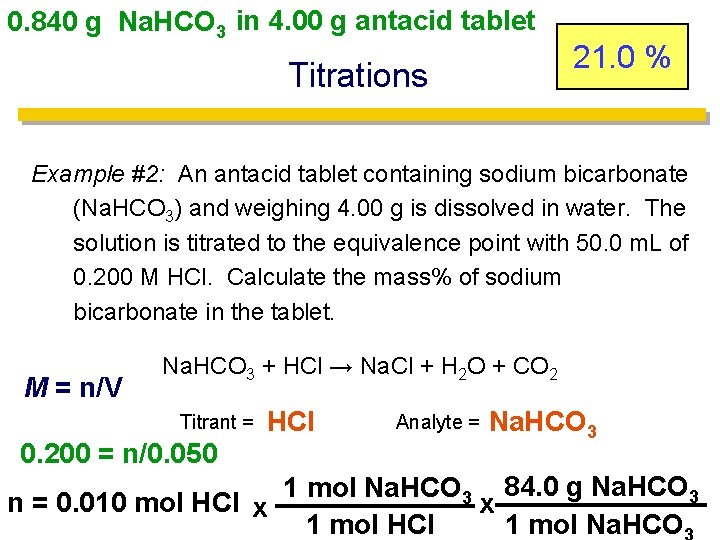# Solution Stoichiometry Solution Stoichiometry uses molarity as a

• Slides: 8Solution Stoichiometry • Solution Stoichiometry uses molarity as a conversion factor between volume and moles of a substance in a solution.Solution Stoichiometry • Titration: • A technique for determining the concentration of a solution.Titrations Titration: the process of analyzing composition by measuring the volume of one solution needed to completely react with another solution. This is a special case of a Limiting Reagent! Usually the reaction of an acid with a base.Titrations Analyte: the solution of unknown concentration but known volume. Titrant: the solution of known concentration. Analyte + Titrant → Products Add titrant until all of the analyte has reacted, then detect the excess of titrant.Titrations Equivalence Point: the point at which exactly the right volume of titrant has been added to complete the reaction. Indicator: substance that changes color when an excess of titrant has been added (phenolphthalein, bromocresol green).Titrations Titration Calculations: 1. Find the number of moles of titrant added to reach the equivalence point. 2. Determine the moles of analyte that must have been present (use stoichiometric coefficients). 3. Determine the concentration of analyte that must have been present in the flask (use the volume of analyte). 4. Calculate the concentration of analyte in the original sample.1 mol HCl 0. 675 mol Na. OH = 0. 025 m. L x x 1 mol Na. OH L Titrations 0. 017 mol HCl in 14. 84 m. L m Example #1: 14. 84 m. L of an HCl solution of unknown concentration is titrated with standard Na. OH solution. At the equivalence point, 25. 0 m. L of the 0. 675 M Na. OH has been added. Calculate the concentration of the HCl solution. Na. OH + HCl → Na. Cl + H 2 O Titrant = ? Na. OH Analyte = ? HCl MA V A = MB V B 1. 14 M0. 840 g Na. HCO 3 in 4. 00 g antacid tablet Titrations 21. 0 % Example #2: An antacid tablet containing sodium bicarbonate (Na. HCO 3) and weighing 4. 00 g is dissolved in water. The solution is titrated to the equivalence point with 50. 0 m. L of 0. 200 M HCl. Calculate the mass% of sodium bicarbonate in the tablet. M = n/V Na. HCO 3 + HCl → Na. Cl + H 2 O + CO 2 Titrant = ? HCl 0. 200 = n/0. 050 Analyte = ? Na. HCO 3 1 mol Na. HCO 3 84. 0 g Na. HCO 3 n = 0. 010 mol HCl x x 1 mol Na. HCO 1 mol HCl